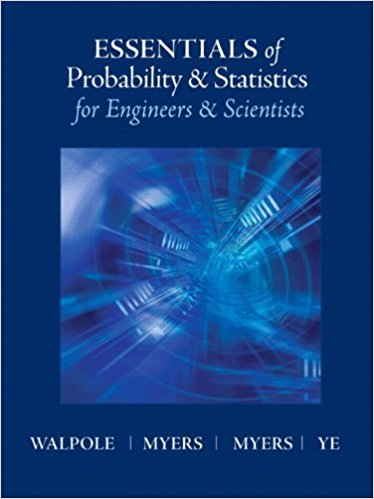×
×

# Solutions for Chapter 7: Linear Regression## Full solutions for Essentials of Probability & Statistics for Engineers & Scientists | 1st Edition

ISBN: 9780321783738Solutions for Chapter 7: Linear Regression

Solutions for Chapter 7
4 5 0 347 Reviews
24
0
##### ISBN: 9780321783738

Chapter 7: Linear Regression includes 93 full step-by-step solutions. Since 93 problems in chapter 7: Linear Regression have been answered, more than 16394 students have viewed full step-by-step solutions from this chapter. This expansive textbook survival guide covers the following chapters and their solutions. Essentials of Probability & Statistics for Engineers & Scientists was written by and is associated to the ISBN: 9780321783738. This textbook survival guide was created for the textbook: Essentials of Probability & Statistics for Engineers & Scientists, edition: 1.

Key Statistics Terms and definitions covered in this textbook
• `-error (or `-risk)

In hypothesis testing, an error incurred by rejecting a null hypothesis when it is actually true (also called a type I error).

• Analysis of variance (ANOVA)

A method of decomposing the total variability in a set of observations, as measured by the sum of the squares of these observations from their average, into component sums of squares that are associated with speciic deined sources of variation

• Bimodal distribution.

A distribution with two modes

• Categorical data

Data consisting of counts or observations that can be classiied into categories. The categories may be descriptive.

• Central tendency

The tendency of data to cluster around some value. Central tendency is usually expressed by a measure of location such as the mean, median, or mode.

• Coeficient of determination

See R 2 .

• Components of variance

The individual components of the total variance that are attributable to speciic sources. This usually refers to the individual variance components arising from a random or mixed model analysis of variance.

• Conditional probability

The probability of an event given that the random experiment produces an outcome in another event.

• Conditional probability density function

The probability density function of the conditional probability distribution of a continuous random variable.

• Conditional variance.

The variance of the conditional probability distribution of a random variable.

• Correlation coeficient

A dimensionless measure of the linear association between two variables, usually lying in the interval from ?1 to +1, with zero indicating the absence of correlation (but not necessarily the independence of the two variables).

• Defect concentration diagram

A quality tool that graphically shows the location of defects on a part or in a process.

• Deining relation

A subset of effects in a fractional factorial design that deine the aliases in the design.

• Deming’s 14 points.

A management philosophy promoted by W. Edwards Deming that emphasizes the importance of change and quality

• Designed experiment

An experiment in which the tests are planned in advance and the plans usually incorporate statistical models. See Experiment

• Eficiency

A concept in parameter estimation that uses the variances of different estimators; essentially, an estimator is more eficient than another estimator if it has smaller variance. When estimators are biased, the concept requires modiication.

• Fisher’s least signiicant difference (LSD) method

A series of pair-wise hypothesis tests of treatment means in an experiment to determine which means differ.

• Fraction defective

In statistical quality control, that portion of a number of units or the output of a process that is defective.

• Fractional factorial experiment

A type of factorial experiment in which not all possible treatment combinations are run. This is usually done to reduce the size of an experiment with several factors.

• Generator

Effects in a fractional factorial experiment that are used to construct the experimental tests used in the experiment. The generators also deine the aliases.

×